# RD Sharma Solutions Class 9 Maths Chapter 25 Probability Exercise 25.1

Probability can range in between 0 to 1, where 0 probability means the event to be an impossible and probability 1 indicates the certain event. Before we get into depth, we should know some basic terms as listed below:

Sample Space- The set of all the possible outcomes to occur in any trial is known as sample space.

Examples- Tossing a coin, Sample Space (S) = {H,T} and Rolling a die, Sample Space (S) = {1,2,3,4,5,6}

Sample Space is made up of Sample Points. Whereas, sample point is one of the possible results. For example, in a deck of cards, 3 of diamond is a sample point

Experiment or Trial- It is a series of action where the outcomes are always uncertain.

Event- It is a single outcome of an experiment.

Learn about the concept in depth and how to solve problems through RD Sharma solutions that are provided at BYJU’S. It acts as perfect self-help guidance for students without any doubt.

## Download PDF of RD Sharma Solutions for Class 9 Maths Chapter 25 Probability Exercise 25.1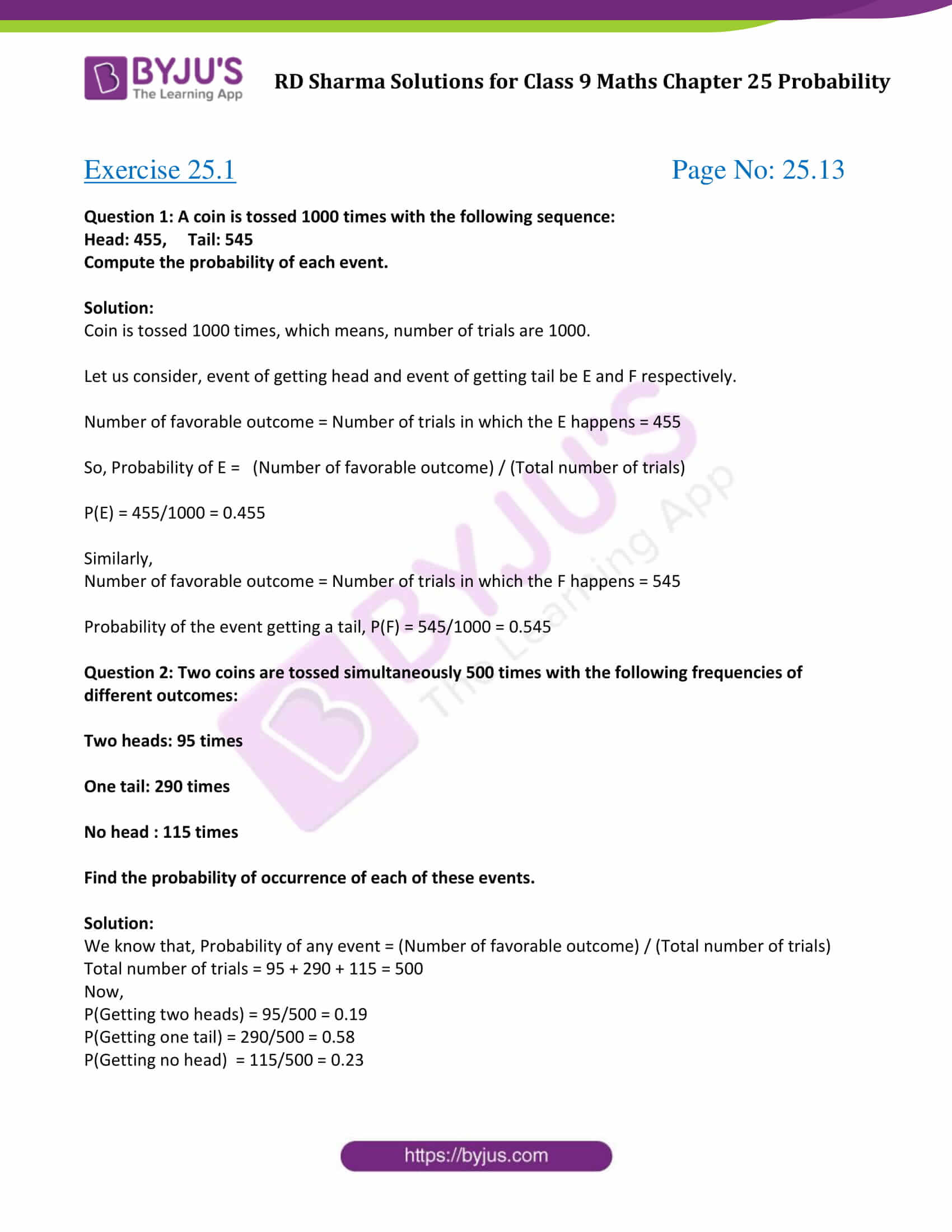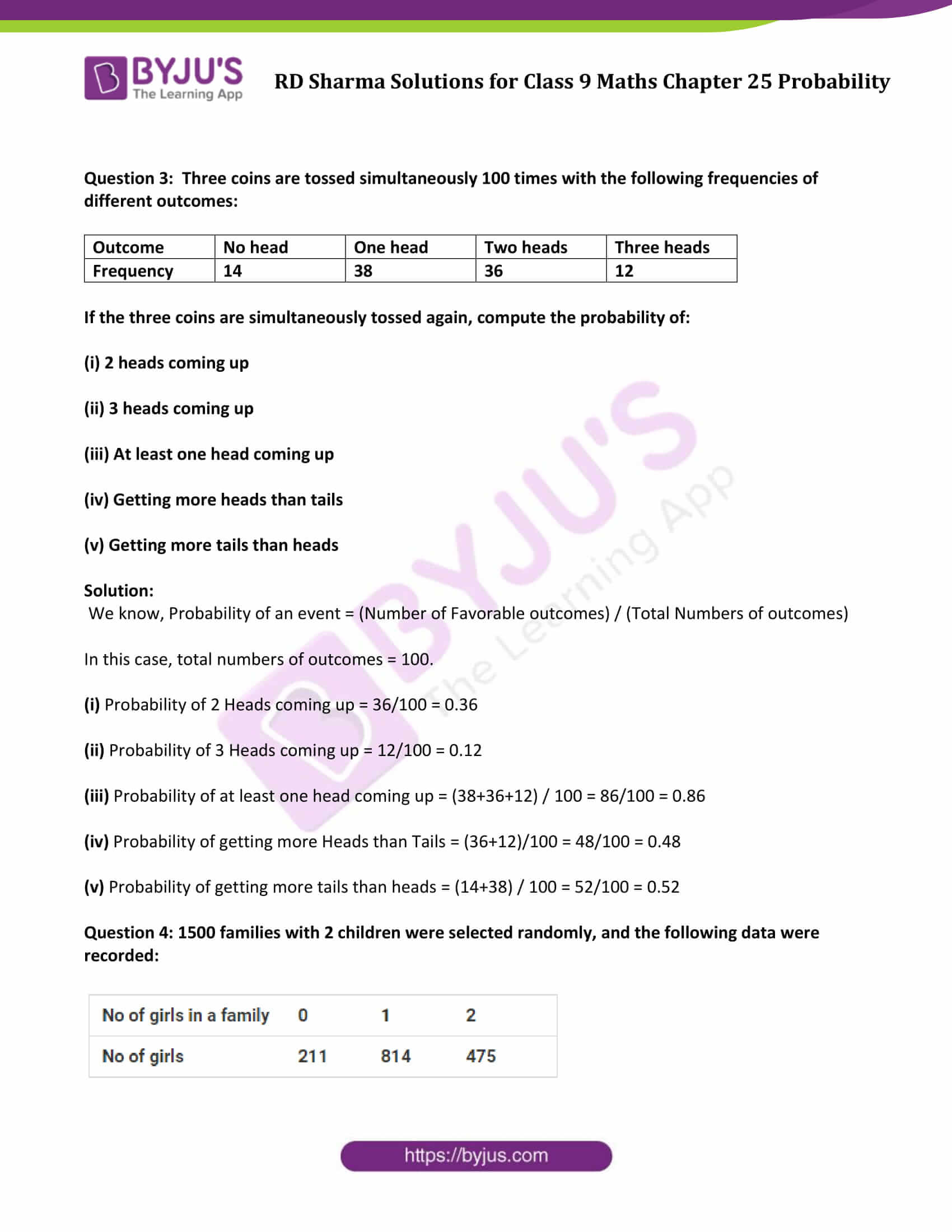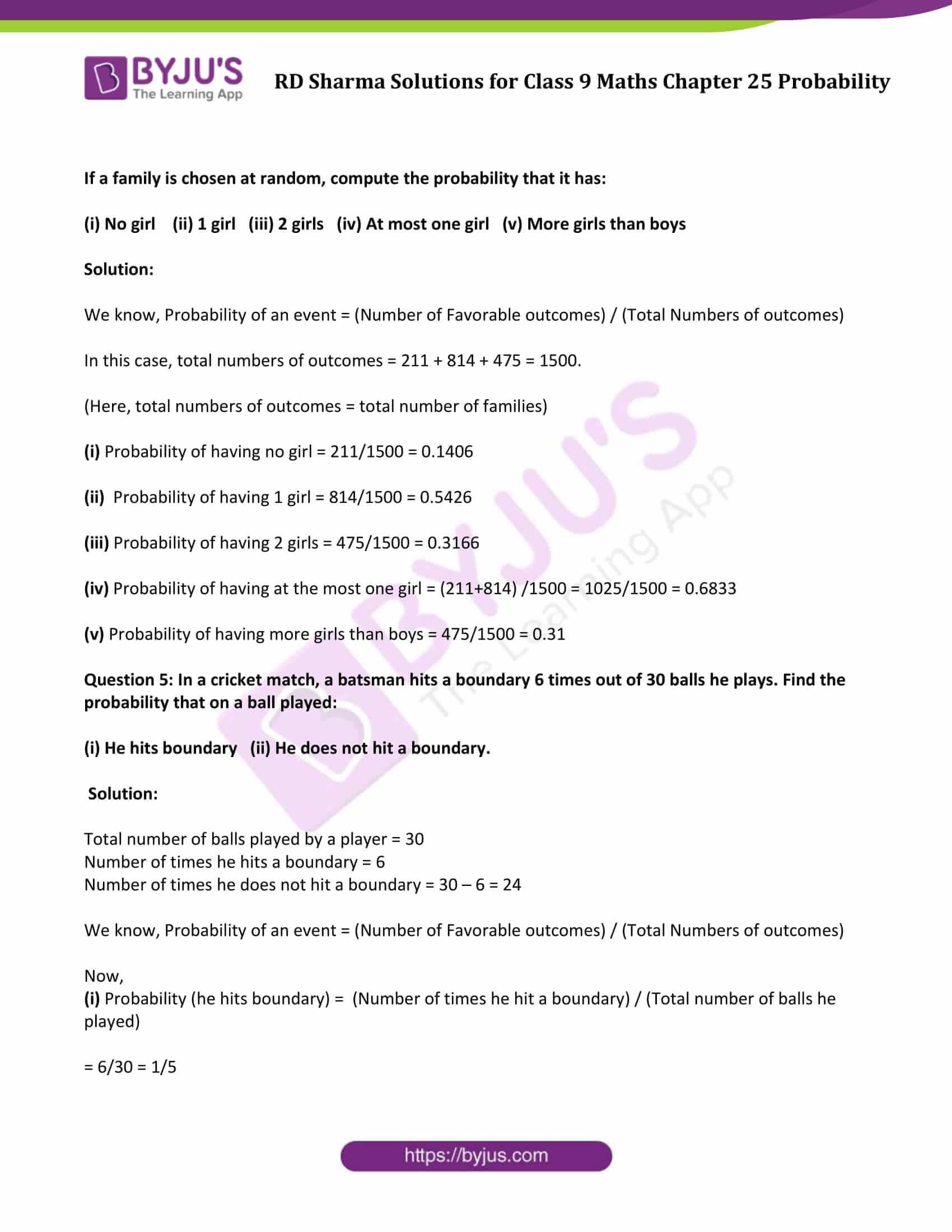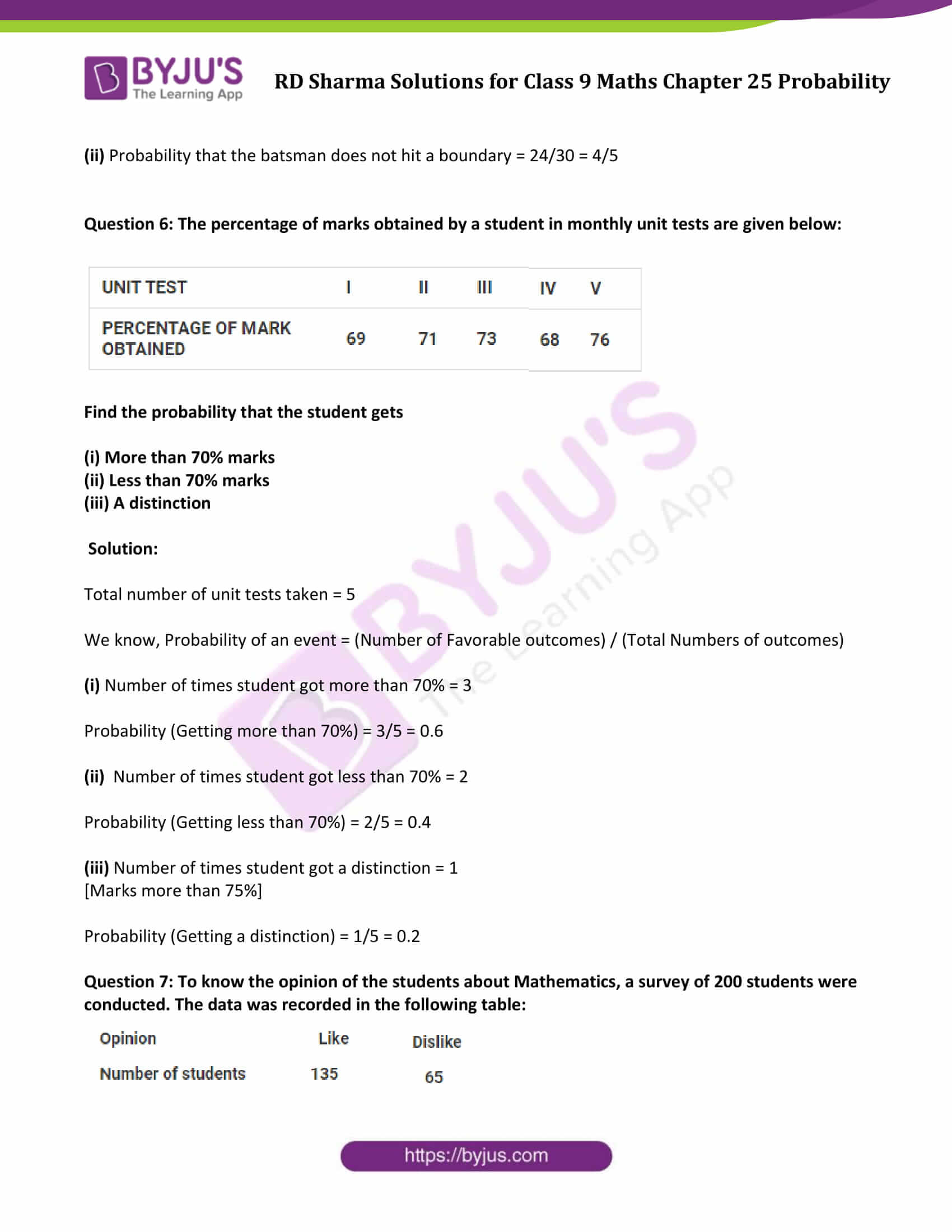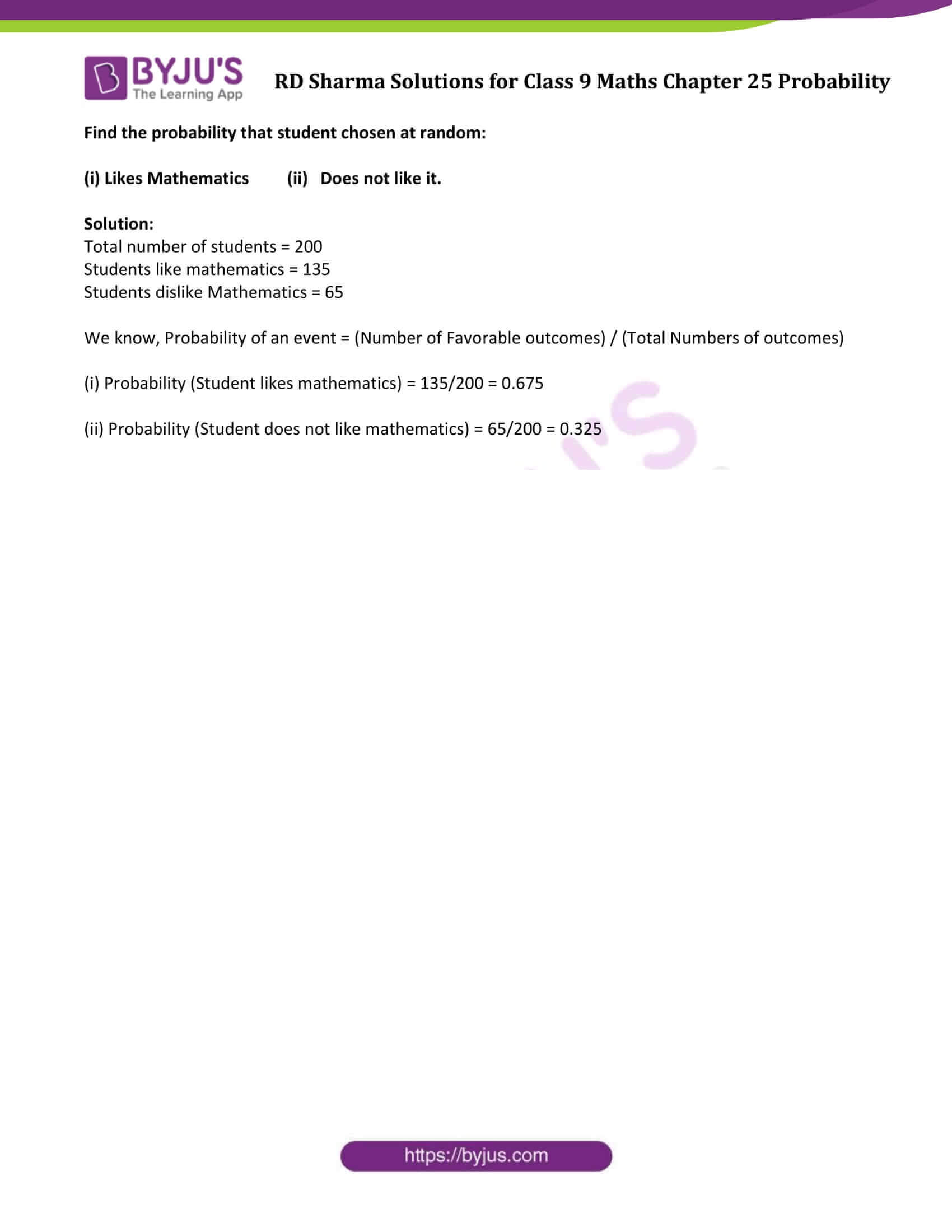### Access Answers to Maths RD Sharma Solutions for Class 9 Chapter 25 Probability Exercise 25.1 Page number 25.13

Exercise 25.1 Page No: 25.13

Question 1: A coin is tossed 1000 times with the following sequence:

Compute the probability of each event.

Solution:

Coin is tossed 1000 times, which means, number of trials are 1000.

Let us consider, event of getting head and event of getting tail be E and F respectively.

Number of favorable outcome = Number of trials in which the E happens = 455

So, Probability of E = (Number of favorable outcome) / (Total number of trials)

P(E) = 455/1000 = 0.455

Similarly,

Number of favorable outcome = Number of trials in which the F happens = 545

Probability of the event getting a tail, P(F) = 545/1000 = 0.545

Question 2: Two coins are tossed simultaneously 500 times with the following frequencies of different outcomes:

One tail: 290 times

Find the probability of occurrence of each of these events.

Solution:

We know that, Probability of any event = (Number of favorable outcome) / (Total number of trials)

Total number of trials = 95 + 290 + 115 = 500

Now,

P(Getting two heads) = 95/500 = 0.19

P(Getting one tail) = 290/500 = 0.58

P(Getting no head) = 115/500 = 0.23

Question 3: Three coins are tossed simultaneously 100 times with the following frequencies of different outcomes:

If the three coins are simultaneously tossed again, compute the probability of:

(iii) At least one head coming up

(iv) Getting more heads than tails

(v) Getting more tails than heads

Solution:

We know, Probability of an event = (Number of Favorable outcomes) / (Total Numbers of outcomes)

In this case, total numbers of outcomes = 100.

(i) Probability of 2 Heads coming up = 36/100 = 0.36

(ii) Probability of 3 Heads coming up = 12/100 = 0.12

(iii) Probability of at least one head coming up = (38+36+12) / 100 = 86/100 = 0.86

(iv) Probability of getting more Heads than Tails = (36+12)/100 = 48/100 = 0.48

(v) Probability of getting more tails than heads = (14+38) / 100 = 52/100 = 0.52

Question 4: 1500 families with 2 children were selected randomly, and the following data were recorded: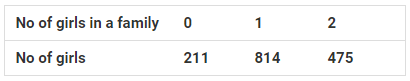If a family is chosen at random, compute the probability that it has:

(i) No girl (ii) 1 girl (iii) 2 girls (iv) At most one girl (v) More girls than boys

Solution:

We know, Probability of an event = (Number of Favorable outcomes) / (Total Numbers of outcomes)

In this case, total numbers of outcomes = 211 + 814 + 475 = 1500.

(Here, total numbers of outcomes = total number of families)

(i) Probability of having no girl = 211/1500 = 0.1406

(ii) Probability of having 1 girl = 814/1500 = 0.5426

(iii) Probability of having 2 girls = 475/1500 = 0.3166

(iv) Probability of having at the most one girl = (211+814) /1500 = 1025/1500 = 0.6833

(v) Probability of having more girls than boys = 475/1500 = 0.31

Question 5: In a cricket match, a batsman hits a boundary 6 times out of 30 balls he plays. Find the probability that on a ball played:

(i) He hits boundary (ii) He does not hit a boundary.

Solution:

Total number of balls played by a player = 30

Number of times he hits a boundary = 6

Number of times he does not hit a boundary = 30 – 6 = 24

We know, Probability of an event = (Number of Favorable outcomes) / (Total Numbers of outcomes)

Now,

(i) Probability (he hits boundary) = (Number of times he hit a boundary) / (Total number of balls he played)

= 6/30 = 1/5

(ii) Probability that the batsman does not hit a boundary = 24/30 = 4/5

Question 6: The percentage of marks obtained by a student in monthly unit tests are given below: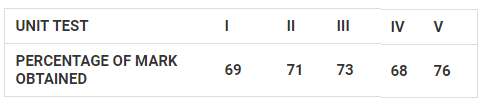Find the probability that the student gets

(i) More than 70% marks

(ii) Less than 70% marks

(iii) A distinction

Solution:

Total number of unit tests taken = 5

We know, Probability of an event = (Number of Favorable outcomes) / (Total Numbers of outcomes)

(i) Number of times student got more than 70% = 3

Probability (Getting more than 70%) = 3/5 = 0.6

(ii) Number of times student got less than 70% = 2

Probability (Getting less than 70%) = 2/5 = 0.4

(iii) Number of times student got a distinction = 1

[Marks more than 75%]

Probability (Getting a distinction) = 1/5 = 0.2

Question 7: To know the opinion of the students about Mathematics, a survey of 200 students were conducted. The data was recorded in the following table: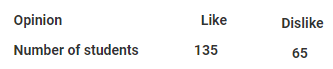Find the probability that student chosen at random:

(i) Likes Mathematics (ii) Does not like it.

Solution:

Total number of students = 200

Students like mathematics = 135

Students dislike Mathematics = 65

We know, Probability of an event = (Number of Favorable outcomes) / (Total Numbers of outcomes)

(i) Probability (Student likes mathematics) = 135/200 = 0.675

(ii) Probability (Student does not like mathematics) = 65/200 = 0.325

## RD Sharma Solutions for Class 9 Maths Chapter 25 Probability Exercise 25.1

RD Sharma Solutions Class 9 Maths Chapter 25 Probability Exercise 25.1 is based on following topics:

• Various approaches to probability
• Experimental or empirical; approach to probability
• Some important terms: Trial, elementary event and compound event